Related Articles
Python | forget_pack() and forget_grid() method in Tkinter
• Last Updated : 07 Jun, 2019

If we want to unmap any widget from the screen or toplevel then` forget()` method is used. There are two types of forget method `forget_pack()` ( similar to `forget() `) and `forget_grid()` which are used with `pack()` and `grid()` method respectively.

### `forget_pack()` method –

```Syntax: widget.forget_pack()

widget can be any valid widget which is visible.
```
Code #1:
 `# Imports tkinter and ttk module``from` `tkinter ``import` `*` `from` `tkinter.ttk ``import` `*`` ` `# toplevel window``root ``=` `Tk()`` ` `# method to make widget invisible``# or remove from toplevel``def` `forget(widget):`` ` `    ``# This will remove the widget from toplevel``    ``# basically widget do not get deleted``    ``# it just becomes invisible and loses its position``    ``# and can be retrieve``    ``widget.forget()`` ` `# method to make widget visible``def` `retrieve(widget):``    ``widget.pack(fill ``=` `BOTH, expand ``=` `True``)`` ` `# Button widgets``b1 ``=` `Button(root, text ``=` `"Btn 1"``)``b1.pack(fill ``=` `BOTH, expand ``=` `True``)`` ` `# See, in command forget() method is passed``b2 ``=` `Button(root, text ``=` `"Btn 2"``, command ``=` `lambda` `: forget(b1))``b2.pack(fill ``=` `BOTH, expand ``=` `True``)`` ` `# In command retrieve() method is passed``b3 ``=` `Button(root, text ``=` `"Btn 3"``, command ``=` `lambda` `: retrieve(b1))``b3.pack(fill ``=` `BOTH, expand ``=` `True``)`` ` `# infinite loop, interrupted by keyboard or mouse``mainloop()`

Output: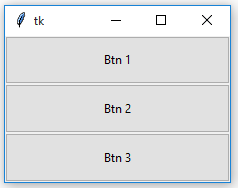After forget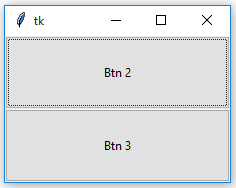After retrieval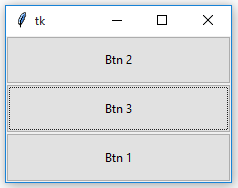Notice the difference in the position of Button 1 before and after forget as well as after retrieval.

### `forget_grid()` method –

```Syntax: widget.forget_grid()

widget can be any valid widget which is visible.
```

Note : This method can be used only with `grid() `geometry methods.

Code #2:

 `# Imports tkinter and ttk module``from` `tkinter ``import` `*``from` `tkinter.ttk ``import` `*`` ` `# toplevel window``root ``=` `Tk()`` ` `# method to make widget invisible``# or remove from toplevel``def` `forget(widget):`` ` `    ``# This will remove the widget from toplevel``    ``# basically widget do not get deleted``    ``# it just becomes invisible and loses its position``    ``# and can be retrieve``    ``widget.grid_forget()`` ` `# method to make widget visible``def` `retrieve(widget):``    ``widget.grid(row ``=` `0``, column ``=` `0``, ipady ``=` `10``, pady ``=` `10``, padx ``=` `5``)`` ` `# Button widgets``b1 ``=` `Button(root, text ``=` `"Btn 1"``)``b1.grid(row ``=` `0``, column ``=` `0``, ipady ``=` `10``, pady ``=` `10``, padx ``=` `5``)`` ` `# See, in command forget() method is passed``b2 ``=` `Button(root, text ``=` `"Btn 2"``, command ``=` `lambda` `: forget(b1))``b2.grid(row ``=` `0``, column ``=` `1``, ipady ``=` `10``, pady ``=` `10``, padx ``=` `5``)`` ` `# In command retrieve() method is passed``b3 ``=` `Button(root, text ``=` `"Btn 3"``, command ``=` `lambda` `: retrieve(b1))``b3.grid(row ``=` `0``, column ``=` `2``, ipady ``=` `10``, pady ``=` `10``, padx ``=` `5``)`` ` `# infinite loop, interrupted by keyboard or mouse``mainloop()`

Output: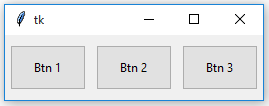After Forget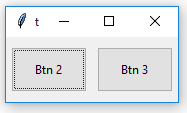After Retrieval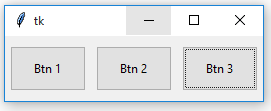Notice that the position of Button 1 remains same after forget and retrieval. With `grid_forget()` method, you can place it at any grid after retrieval but generally, the original grid is chosen.

Attention geek! Strengthen your foundations with the Python Programming Foundation Course and learn the basics.

To begin with, your interview preparations Enhance your Data Structures concepts with the Python DS Course. And to begin with your Machine Learning Journey, join the Machine Learning – Basic Level Course

My Personal Notes arrow_drop_up